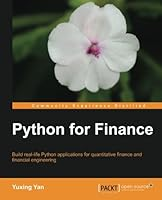# Python for Finance7 Reviews
2014-05-11
408 pages

## Book Description

Build real-life applications for quantitative finance and engineering with this book and ebook

Overview

• Estimate market risk, form various portfolios, and estimate their variance-covariance matrixes using real-world data
• Explains many financial concepts and trading strategies with the help of graphs
• A step-by-step tutorial with many Python programs that will help you learn how to apply Python to finance

In Detail

Python is a free and powerful tool that can be used to build a financial calculator and price options, and can also explain many trading strategies and test various hypotheses. This book details the steps needed to retrieve time series data from different public data sources.

Python for Finance explores the basics of programming in Python. It is a step-by-step tutorial that will teach you, with the help of concise, practical programs, how to run various statistic tests. This book introduces you to the basic concepts and operations related to Python. You will also learn how to estimate illiquidity, Amihud (2002), liquidity measure, Pastor and Stambaugh (2003), Roll spread (1984), spread based on high-frequency data, beta (rolling beta), draw volatility smile and skewness, and construct a binomial tree to price American options.

This book is a hands-on guide with easy-to-follow examples to help you learn about option theory, quantitative finance, financial , and time series using Python.

What you will learn from this book

• Build a financial calculator based on Python
• Learn how to price various types of options such as European, American, average, lookback, and barrier options
• Estimate returns and convert daily returns into monthly or annual returns
• Form an n-stock portfolio and estimate its variance-covariance matrix
• Estimate VaR (Value at Risk) for a stock or portfolio
• Run CAPM (Capital Asset Pricing Model) and the Fama- 3-factor model
• Learn how to optimize a portfolio and draw an efficient frontier
• Conduct various statistic tests such as T-tests, F-tests, and normality tests

Approach

Chapter 1: Introduction and Installation of Python
Chapter 2: Using Python as an Ordinary Calculator
Chapter 3: Using Python as a Financial Calculator
Chapter 4: 13 Lines of Python to Price a Call Option
Chapter 5: Introduction to
Chapter 6: Introduction to NumPy and SciPy
Chapter 7: Visual Finance via Matplotlib
Chapter 8: of Time Series
Chapter 9: The Black-Scholes-Merton Option Model
Chapter 10: Python Loops and Implied Volatility
Chapter 11: Monte Carlo Simulation and Options
Chapter 12: Volatility Measures and GARCH

## Book Details

• Title: Python for Finance
• Author:
• Length: 408 pages
• Edition: 1
• Language: English
• Publisher:
• Publication Date: 2014-05-11
• ISBN-10: 1783284374
• ISBN-13: 9781783284375